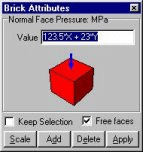# Building finite element models in Strand7

 General equation input Enter numbers as mathematical expressions. For example, you can assign node forces and plate pressures as functions of position. The application of a hydrostatic pressure in the Y direction can be easily applied by entering equations such as "1000*9.81*(Y-23.4)". Variables in any coordinate system may be used.